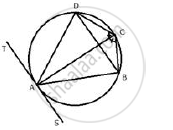Share

In a Cyclic Quadrilateral Abcd, the Diagonal Ac Bisects the Angle Bcd. Prove that the Diagonal Bd is Parallel to the Tangent to the Circle at Point A. - ICSE Class 10 - Mathematics

Question

In a cyclic quadrilateral ABCD, the diagonal AC bisects the angle BCD. Prove that the diagonal BD is parallel to the tangent to the circle at point A.

Solution∠ADB = ∠ACB …… (i) (Angles in same segement)
Similarly ,
∠ABD = ∠ACD …….. (ii)

But, ∠ACB = ∠ACD (AC is bisector of ∠BCD)
∴ ∠ADB = ∠ABD (from (i) and (ii) )
TAS is a tangent and AB is a chord
∴ ∠BAS =  ∠ADB (angles in alternate segment)
But, ∠ADB = ∠ABD
∴ ∠BAS = ∠ABD
But these are alternate angles
Therefore, TS ∥ BD

Is there an error in this question or solution?

APPEARS IN

Solution In a Cyclic Quadrilateral Abcd, the Diagonal Ac Bisects the Angle Bcd. Prove that the Diagonal Bd is Parallel to the Tangent to the Circle at Point A. Concept: Cyclic Properties.
S